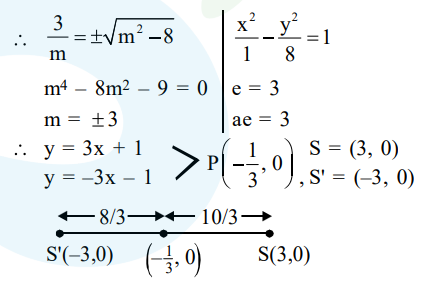# Let P be the point of intersection of the common tangents

Question:

Let $P$ be the point of intersection of the common tangents to the parabola $\mathrm{y}^{2}=12 \mathrm{x}$ and the hyperbola $8 x^{2}-y^{2}=8$. If $S$ and $S^{\prime}$ denote the foci of the hyperbola where $S$ lies on the positive $x$-axis then $P$ divides $\mathrm{SS}^{\prime}$ in a ratio:

1. $5: 4$

2. $14: 13$

3. $2: 1$

4. $13: 11$

Correct Option: 1

Solution:

Equation of tangents

$\mathrm{y}^{2}=12 \mathrm{x} \quad \Rightarrow \mathrm{y}=2 \mathrm{x}+\frac{3}{\mathrm{~m}}$

$\frac{x^{2}}{1}-\frac{y^{2}}{8}=1 \Rightarrow y=m x \pm \sqrt{m^{2}-8}$

Since they are common tangent